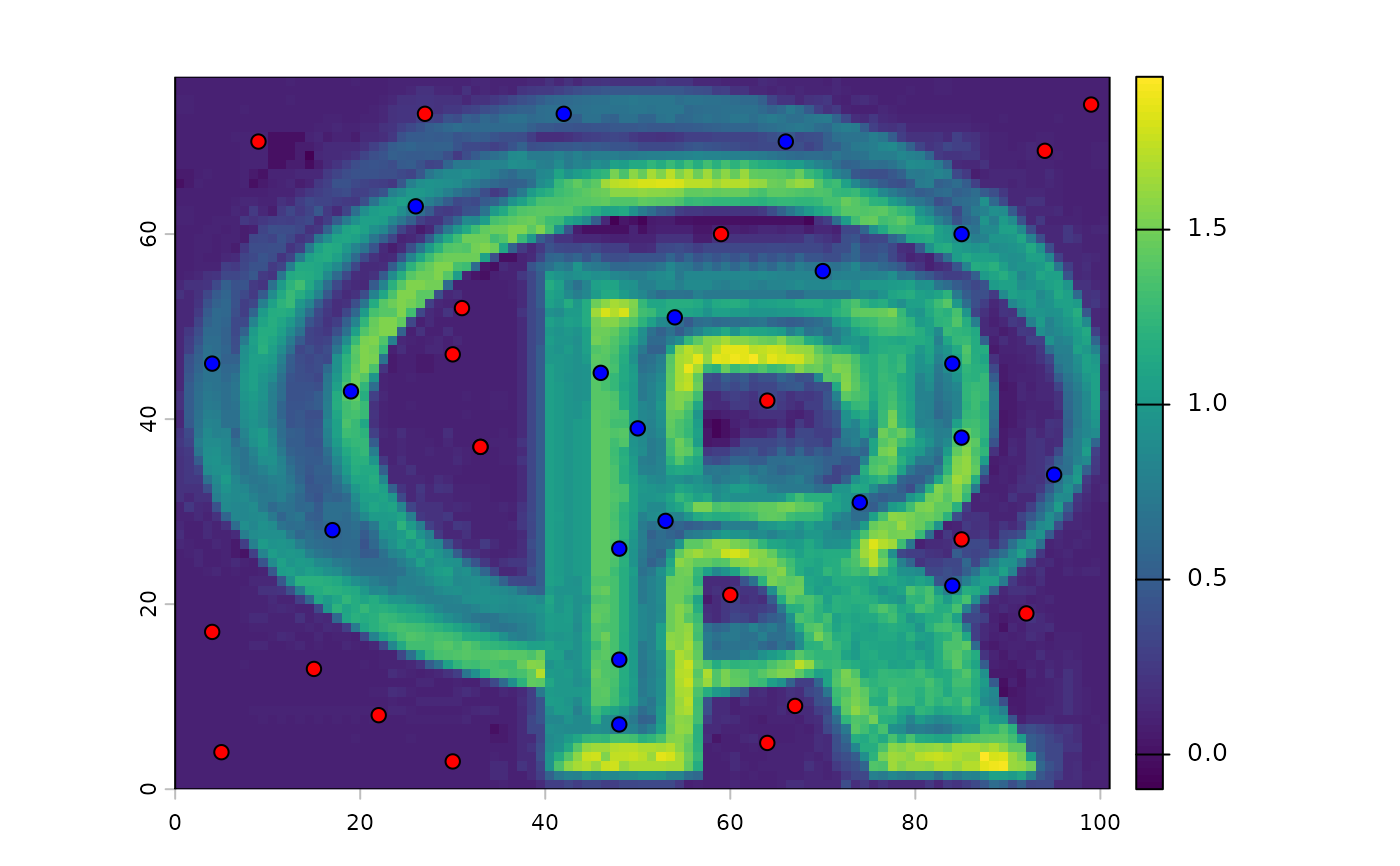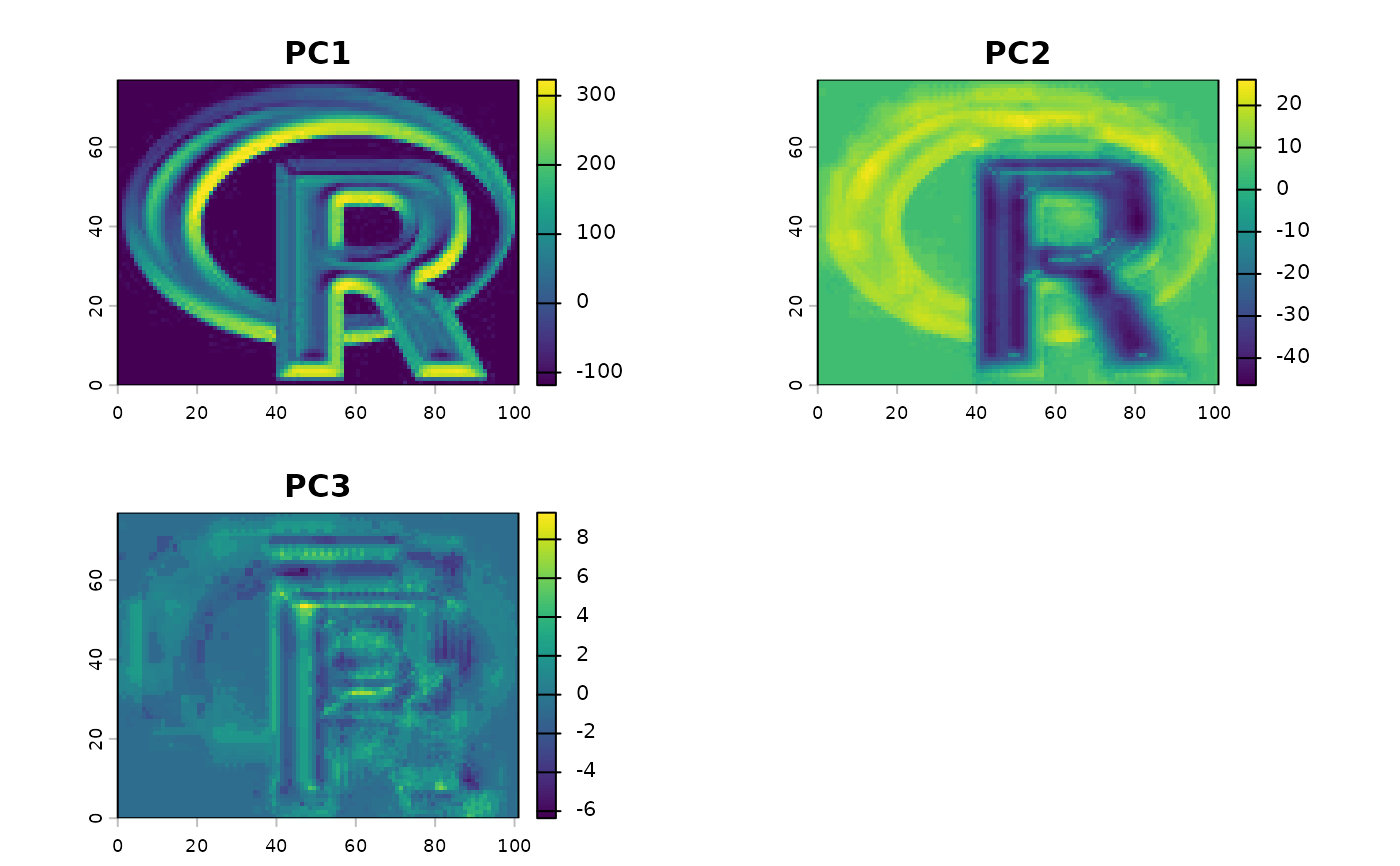Make a SpatRaster object with predictions from a fitted model object (for example, obtained with glm or randomForest). The first argument is a SpatRaster object with the predictor variables. The names in the Raster object should exactly match those expected by the model. Any regression like model for which a predict method has been implemented (or can be implemented) can be used.

The method should work if the model's predict function returns a vector, matrix or data.frame (or a list that can be coerced to a data.frame). In other cases it may be necessary to provide a custom "predict" function that wraps the model's predict function to return the values in the required form. See the examples.

This approach of using model predictions is commonly used in remote sensing (for the classification of satellite images) and in ecology, for species distribution modeling.

# S4 method for SpatRaster
predict(object, model, fun=predict, ..., const=NULL, na.rm=FALSE,
index=NULL, cores=1, cpkgs=NULL, filename="", overwrite=FALSE, wopt=list())

## Arguments

object

SpatRaster

model

fitted model of any class that has a "predict" method (or for which you can supply a similar method as fun argument. E.g. glm, gam, or randomForest

fun

function. The predict function that takes model as first argument. The default value is predict, but can be replaced with e.g. predict.se (depending on the type of model), or your own custom function

...

additional arguments for fun

const

data.frame. Can be used to add a constant value as a predictor variable so that you do not need to make a SpatRaster layer for it

na.rm

logical. If TRUE, cells with NA values in the any of the layers of x are removed from the computation (even if the NA cell is in a layer that is not used as a variable in the model). This option prevents errors with models that cannot handle NA values when makeing predictions. In most other cases this will not affect the output. However, there are some models that return predicted values even if some (or all) variables are NA

index

integer. To select subset of output variables

cores

positive integer. If cores > 1, a 'parallel' package cluster with that many cores is created and used

cpkgs

character. The package(s) that need to be loaded on the nodes to be able to run the model.predict function (see examples)

filename

character. Output filename

overwrite

logical. If TRUE, filename is overwritten

wopt

list with named options for writing files as in writeRaster

## Value

SpatRaster

interpolate for spatial model prediction

## Examples

logo <- rast(system.file("ex/logo.tif", package="terra"))
names(logo) <- c("red", "green", "blue")
p <- matrix(c(48, 48, 48, 53, 50, 46, 54, 70, 84, 85, 74, 84, 95, 85,
66, 42, 26, 4, 19, 17, 7, 14, 26, 29, 39, 45, 51, 56, 46, 38, 31,
22, 34, 60, 70, 73, 63, 46, 43, 28), ncol=2)

a <- matrix(c(22, 33, 64, 85, 92, 94, 59, 27, 30, 64, 60, 33, 31, 9,
99, 67, 15, 5, 4, 30, 8, 37, 42, 27, 19, 69, 60, 73, 3, 5, 21,
37, 52, 70, 74, 9, 13, 4, 17, 47), ncol=2)

xy <- rbind(cbind(1, p), cbind(0, a))

# extract predictor values for points
e <- extract(logo, xy[,2:3])

# combine with response (excluding the ID column)
v <- data.frame(cbind(pa=xy[,1], e))

#build a model, here with glm
model <- glm(formula=pa~., data=v)

#predict to a raster
r1 <- predict(logo, model)

plot(r1)
points(p, bg='blue', pch=21)
points(a, bg='red', pch=21)# logistic regression
model <- glm(formula=pa~., data=v, family="binomial")
#> Warning: glm.fit: algorithm did not converge
#> Warning: glm.fit: fitted probabilities numerically 0 or 1 occurred
r1log <- predict(logo, model, type="response")

# to get the probability and standard error
r1se <- predict(logo, model, se.fit=TRUE)

# or provide a custom predict function

predfun <- function(model, data) {
v <- predict(model, data, se.fit=TRUE)
cbind(p=as.vector(v$fit), se=as.vector(v$se.fit))
}

r2 <- predict(logo, model, fun=predfun)

# principal components of a SpatRaster
pca <- prcomp(logo)

# or use sampling if cannot process all cell values
sr <- spatSample(logo, 100000, "regular")
pca <- prcomp(sr)

x <- predict(logo, pca)
plot(x)## parallelization
if (FALSE) {
## simple case with GLM
model <- glm(formula=pa~., data=v)
p <- predict(logo, model, cores=2)

## The above does not work with a model from a contributed
## package, as the package needs to be loaded in each core.
## Below are three approaches to deal with that

library(randomForest)
rfm <- randomForest(formula=pa~., data=v)

## approach 0 (not parallel)
rp0 <- predict(logo, rfm)

## approach 1, use the "cpkgs" argument
rp1 <- predict(logo, rfm, cores=2, cpkgs="randomForest")

## approach 2, write a custom predict function that loads the package
rfun <- function(mod, dat, ...) {
library(randomForest)
predict(mod, dat, ...)
}
rp2 <- predict(logo, rfm, fun=rfun, cores=2)

## approach 3, write a parallelized custom predict function
rfun <- function(mod, dat, ...) {
ncls <- length(cls)
nr <- nrow(dat)
s <- split(dat, rep(1:ncls, each=ceiling(nr/ncls), length.out=nr))
unlist(  parallel::clusterApply(cls, s, function(x, ...) predict(mod, x, ...))  )
}

library(parallel)
cls <- parallel::makeCluster(2)
parallel::clusterExport(cls, c("rfm", "rfun", "randomForest"))
rp3 <- predict(logo, rfm, fun=rfun)
parallel::stopCluster(cls)

plot(c(rp0, rp1, rp2, rp3))

### with two output variables (probabilities for each class)
v$pa <- as.factor(v$pa)
rfm2 <- randomForest(formula=pa~., data=v)
rfp <- predict(logo, rfm2, cores=2, type="prob", cpkgs="randomForest")
}